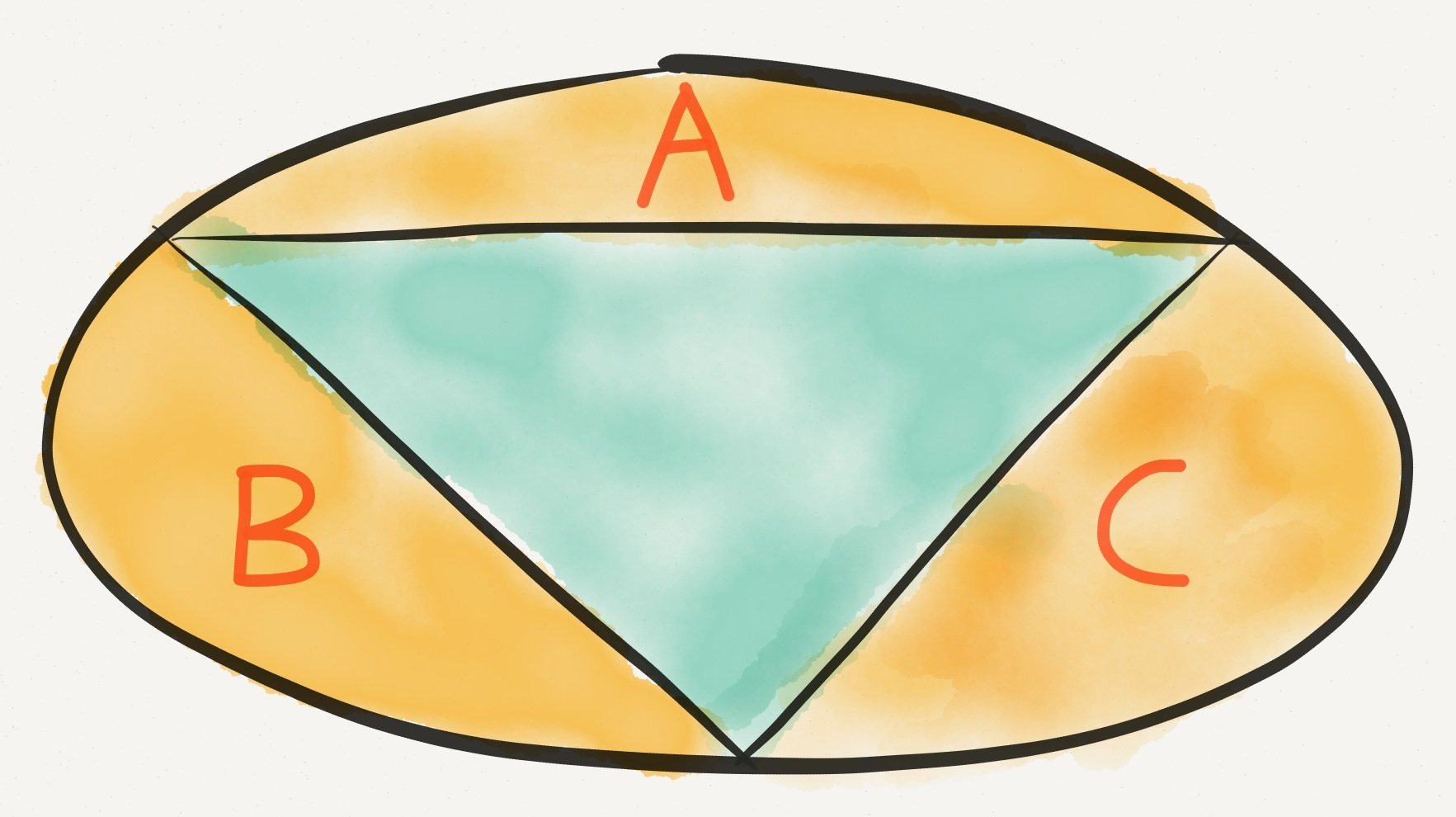# Day 22: Ellipse or Stretched Circle?

Geometry Level 4A triangle splits an ellipse into four sections. The three parts that have a curve (shown in the diagram) are of equal area ($A=B=C$).

Also, the ellipse is three times as long as it is wide, and one side of the triangle is parallel to its major axis.

What is the obtuse angle in the triangle?

×